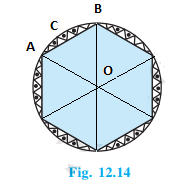Newbie

# A round table cover has six equal designs as shown in Fig. 12.14. If the radius of the cover is 28 cm, find the cost of making the designs at the rate of ₹ 0.35 per cm2 . (Use √3 = 1.7).Q.13

• 0

Give me the solution of ncert class 10 areas related to circles of exercise 12.2 question no. 13 please give me the solution of this question Find the best and easiest way to solve this question.A round table cover has six equal designs as shown in Fig. 12.14. If the radius of the cover is 28 cm, find the cost of making the designs at the rate of ₹ 0.35 per cm2 . (Use √3 = 1.7).

Share

1.Total number of equal designs = 6

AOB= 360°/6 = 60°

Radius of the cover = 28 cm

Cost of making design = ₹ 0.35 per cm2

Since the two arms of the triangle are the radii of the circle and thus are equal, and one angle is 60°, ΔAOB is an equilateral triangle. So, its area will be (√3/4)×a2 sq. units

Here, a = OA

∴ Area of equilateral ΔAOB = (√3/4)×28= 333.2 cm2

Area of sector ACB = (60°/360°)×πrcm2

= 410.66 cm2

So, area of a single design = area of sector ACB – area of ΔAOB

= 410.66 cm2 – 333.2 cm= 77.46 cm2

∴ Area of 6 designs = 6×77.46 cm= 464.76 cm2

So, total cost of making design = 464.76 cm×Rs.0.35 per cm2

= Rs. 162.66

• 0